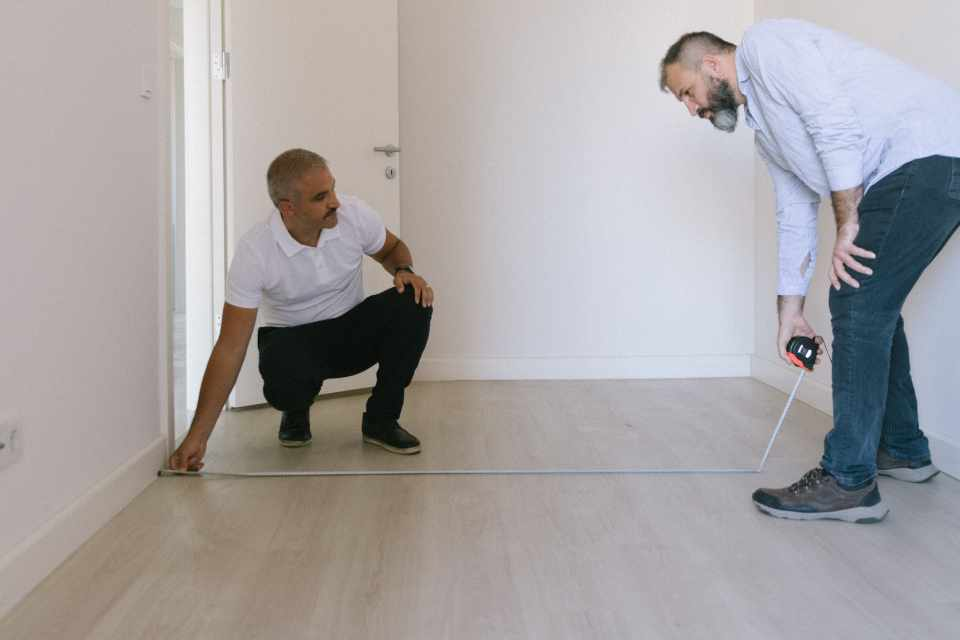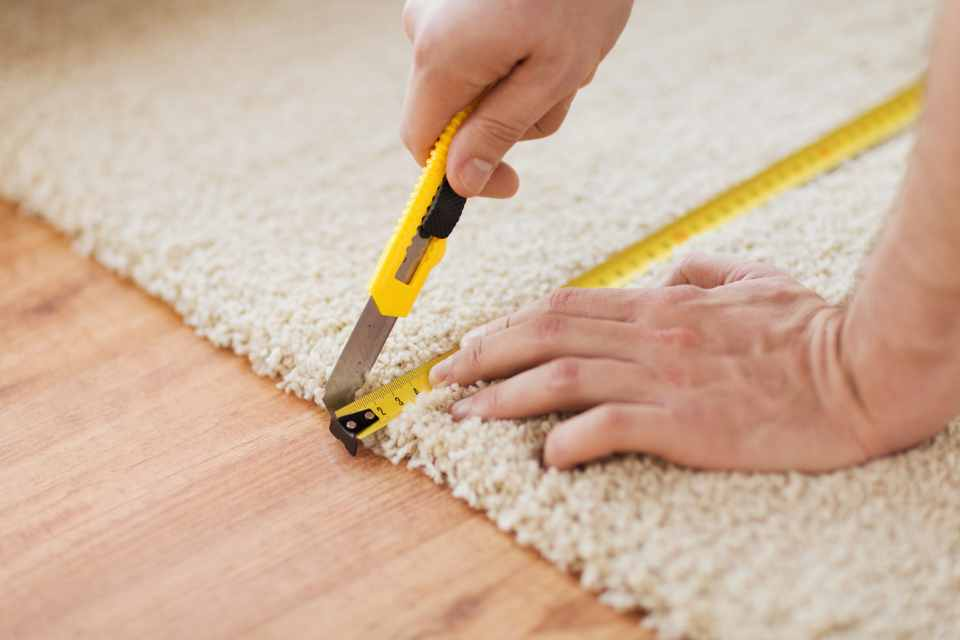# Guide to Calculating Square Footage

When planning for a new flooring project, one of the very first steps you should take as a homeowner is to accurately calculate the square footage of the rooms in question. Your budget will thank you in advance! By having the total square footage handy, you can more quickly determine if the stunning floors that have caught your eye are a realistic option for your home and your budget.Of course, there is more to determining your flooring cost than simply measuring the length and width of a room, but learning how to calculate the square feet of the living space you're looking to renovate is a good start! And don’t be afraid if your math skills aren't up to par, as we've made our square footage calculator guide easy enough for anyone to follow. Here are five simple steps on how to calculate flooring square footage like a pro and accurately assess your project:

## Step 1

Start by gathering measurements of the rooms where you're going to install new flooring. Use a tape measure or download an easy-measure app on your phone, which will allow you to take measurements of the room with your camera. As you probably anticipated, you will be taking length and width measurements of the room and rounding each measurement up to the nearest inch.

For example: If you measure the length of the room as 12 feet, 6 inches, and 9/16th of an inch, you would round up the total measurement to 12 feet and 7 inches.## Step 2

Convert the measurements that you took in feet and inches to measurements that are strictly in terms of feet. To get the total feet, divide the inches in your measurement by 12 and add that figure to your foot measurement. These totals will be the width and length measurements to use.

For example: If the room is 12 feet and 7 inches in length, you would divide the 7 inches by 12, which equals .583. Add this figure to 12 feet, and your final length is 12.583 feet.

## Step 3

Now, multiply the length of the room by the width of the room to determine the square footage, which of course is expressed in square feet.

For example: If the room is 12.583 ft. in length and 9.5 ft. in width, you would multiply the two figures (12.583 x 9.5) to determine the square footage, which equals 119.54 square feet.

## Step 4

If the room or area you are measuring is not a perfect square or rectangle, such as an L-shape, you can split it up and treat it as two separate square or rectangular areas. Now calculate the area of each section separately and add them together for your total.

## Step 5

You can easily convert a square foot measurement to square yards and just as easily convert back if needed. Simply divide the total square footage by 9 to determine square yardage. Similarly, multiply a square yard measurement by 9 to determine square footage.

For example: If the square footage of a room is 119.54 sq ft., you would divide this figure by 3 (119.54 ÷ 9) to determine the square yardage, which equals 13.28 sq yd.

Once you have determined your actual square footage, it’s wise to allow for at least a 5%–10% overage in flooring material to cover any possible installation mishaps or defects noticed during installation. This overage could also be higher depending on the size and shape of the area you're working with, such as rooms with any tricky inlets or areas with odd dimensions. Laminate flooring, or another type of flooring that can be cut, is an excellent choice to consider if you will need extra material to customize to fit odd dimensions. For carpet installation, it’s best to plan for a 20% overage in flooring material. Add the appropriate overage figure to your square footage, and now you are ready to estimate the flooring cost.For example: If your total square footage is 119.54 sq ft., you can determine that 10% overage is close to 12 sq ft. (120 sq ft. x .10 = 12 sq ft.). Add the overage figure of 12 sq ft. to 119.54 sq ft. to arrive at a total of 131.54 sq ft., which easily rounds up to 132 sq ft. needed for your project.

## FAQ

### What is the formula for calculating area square footage?

Calculating the square footage of a rectangular room is a straightforward process that involves multiplying the length and width of the space. To find the area in square feet, simply measure the length and width of the rectangular room in feet and then multiply these two dimensions. The formula for calculating square footage is area = length × width.

Not all rooms are simple rectangles or squares, which makes the process of calculating square footage a little harder. In such cases, you can break down the irregular shape into smaller, more manageable geometric shapes (such as rectangles, squares, and triangles), calculate the area of each individual shape, and then sum them up to find the total square footage.

### How many square feet is a 12x12 room?

To measure square footage, multiply the length and width of the room in feet. In the case of a 12x12 room, simply multiply 12 by 12, resulting in 144 square feet. This uncomplicated formula provides an efficient way to gauge the area of any space.

## Get Started with Our Free Flooring Estimate

Now that you know how to calculate square footage, what’s your new floor going to be? A stunning all-natural hardwood flooring? A classic marble stone? A waterproof vinyl? Once you’ve selected your favorite flooring product, you can use a square foot calculator (such as the one featured on this page) to estimate your total flooring material cost for that specific product. We suggest picking your top 5–10 favorite flooring products in advance and making a chart that compares the total flooring material cost for each, side by side, as you calculate each of your favorite styles.Now that you have your total flooring material, take a moment to add in any other costs, or factors that must be considered, to your total project budget. These can include whether you will need professional installation, new underlayment or padding, or purchasing a warranty. In addition to flooring material costs, our Associated Costs guide can also help you think of these other factors in your project.

Sometimes it can help to get additional opinions if you feel overwhelmed or experience doubts in your calculations. To receive a total project cost estimate, you can always submit a Free Estimate Request Form to your local Carpet One Floor & Home store or work directly with one of our helpful flooring experts in-store.